# Bihar Board 12th Chemistry Objective Answers Chapter 1 The Solid State

Bihar Board 12th Chemistry Objective Questions and Answers

## Bihar Board 12th Chemistry Objective Answers Chapter 1 The Solid State

Question 1.
Which among the following will show anisotropy ?
(a) Glass
(b) NaBr
(c) Plastic
(d) Rubber
(b) NaBr

Question 2.
Which of the following forms a molecular solid when solidified ?
(a) Calcium fluoride
(b) Silicon dioxide
(c) Carbon dioxide
(d) Sodium chloride
(c) Carbon dioxide

Question 3.
The major binding force in diamond, silicon and quartz is ……………
(a) electrostatic force
(b) electrical attraction
(c) covalent bond force
(d) van der Waals force
(c) covalent bond force

Question 4.
Solid X is a very hard solid which is electrical insulator in solid as well as in molten state and has extremely high melting point. What type of solid is it ?
(a) Ionic solid
(b) Covalent solid
(c) Metallic solid
(d) Molecular solid
(b) Covalent solidQuestion 5.
Examples of few solids are given below. Find out the example which is not correctly matched.
(a) Ionic solids – NaCl, ZnS
(b) Covalent solids – H2,l2
(c) Molecular solids – H2O(s)
(d) Metallic solids – Cu, Sn
(b) Covalent solids – H2,l2

Question 6.
Which of the following solids is the structure of CsCl crystal ?
(a) Body centred cubic
(b) Simple cubic
(c) Face centred cubic
(d) Edge centred cubic
(a) Body centred cubic

Question 7.
Which of the following does not represent a type of crystal system ?
(a) Triclinic
(b) Monoclinic
(c) Rhombohedral
(d) Isotropical
(d) Isotropical

Question 8.
Which of the following primitive cells show the given parameters ?
a # b # c, α = β = Y = 90°
(a) Cubic
(b) Tetragonal
(c) Orthorhombic
(d) Hexagonal
(c) Orthorhombic

Question 9.
Which type of crystals contains more than one Bravais lattice ?
(a) Hexagonal
(b) Triclinic
(c) Rhombohedral
(d) Monoclinic
(d) MonoclinicQuestion 10.
For the structure given below the site marked as S is a ……………
(a) tetrahedral void
(b) cubic void
(c) octahedral void
(d) none of these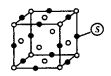(c) octahedral void

Question 11.
A metal crystallises into a lattice containing a sequence of layers as AB AB AB………………….. What percentage of voids are left in the lattice ?
(a) 72%
(b) 48%
(c) 26%
(d) 32%
(c) 26%

Question 12.
In ccp arrangement the pattern of successive layers can be designated as …………
(a) AB AB AB
(b) ABC ABC ABC
(c) AB ABC AB
(d)  ABA ABA ABA
(b) ABC ABC ABC

Question 13.
The coordination number of metal crystallising in a hexagonal close packing is ………
(a) 12
(b) 4
(c) 8
(d) 6
(a) 12

Question 14.
A crystalline structure has radius ratio (r, / r) in the range of 0.225 – 0.414. The coordination number and arrangement of anions around the cations are ………..
(a) 3, plane triangular
(b) 6, octahedral
(c) 4, tetrahedral
(d) 8, cubic
(c) 4, tetrahedralQuestion 15.
A crystal lattice with alternative +ve and -ve ions has radius ratio 0.524. The coordination number of lattice is …………
(a) 4
(b) 6
(c) 8
(d) 12
(b) 6

Question 16.
A solid AB has a rock salt structure. If radius of cation A+ is 120 pm, what is the minimum value of radius of B+anion (a) 120 pm
(b) 240 pm
(c) 290 pm
(d) 360 pm
(c) 290 pmQuestion 17.
How many chloride ions are surrounding sodium ion in sodium chloride crystal ?
(a) 4
(b) 8
(c) 6
(d) 12
(c) 6

Question 18.
In NaCl structure, ……………
(a) all octahedral and tetrahedral sites are occupied
(b) only octahedral sites are occupied
(c) only tetrahedral sites are occupied
(d) neither octahedral nor tetrahedral sites are occupied.
(b) only octahedral sites are occupied

Question 19.
NaCl type crystal (with coordination no. 6 : 6) can be converted into CsCl type crystal (with coordination no. 8 : 8) by applying ……
(a) high temperature
(b) high pressure
(c) high temperature and high pressure
(d) low temperature and low pressure.
(b) high pressureQuestion 20.
Coordination numbers of Cs+ and Cl in CsCl crystal are ………….
(a) 8, 8
(b) 4,4
(c) 6,6
(d) 8,4
(a) 8, 8

Question 21.
A unit cell of BaCl2 (fluorite structure) is made up of ………..
(a) four Ba2+ ions and four Cl ions
(b) four Ba2+ ions and eight Cl ions
(c) eight Ba2+ ions and four Cl ions
(d) four Ba2+ ions and six Cl
(c) eight Ba2+ ions and four Cl ions

Question 22.
In face centred cubic unit cell, edge length is …………..
(a) $$\frac{4}{\sqrt{3}} \mathrm{r}$$
(b) $$\frac{4}{\sqrt{2}} \mathrm{r}$$
(c) 2r
(d) $$\frac{\sqrt{3}}{2} \mathrm{r}$$
(b) $$\frac{4}{\sqrt{2}} \mathrm{r}$$

Question 23.
The edge length of fee cell is 508 pm. If radius of cation is 110 pm, the radius of anion is …………
(a) 110 pm
(b) 220 pm
(c) 285 pm
(d) 144 pm
(d) 144 pm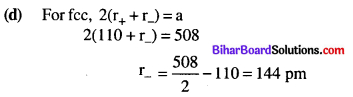Question 24.
The edge length of sodium chloride unit cell is 564 pm. If the size of Cl ion is 181 pm. The size of Na+ ion will be …….
(a) 101 pm
(b) 181 pm
(c) 410 pm
(d) 202 pm
(a) 101 pmQuestion 25.
The radii of Na+ and Cl ions are 95 pm and 181 pm respectively. The edge length of NaCl unit cell is ………..
(a) 276 pm
(b) 138 pm
(c) 552 pm
(d) 415 pm
(c) 552 pm

Question 26.
Copper crystallises in fee with a unit cell length of 361 pm. What is the radius of copper atom ?
(a) 157 pm
(b) 181 pm
(c) 127 pm
(d) 108 pm
(c) 127 pm

Question 27.
Total volume of atoms present in a fee unit cell of a metal with radius r is …………
(a) $$\frac{12}{3} \pi r^{2}$$
(b) $$\frac{16}{3} \pi r^{3}$$
(c) $$\frac{20}{3} \pi r^{3}$$
(d) $$\frac{24}{3} \pi r^{3}$$
(b) $$\frac{16}{3} \pi r^{3}$$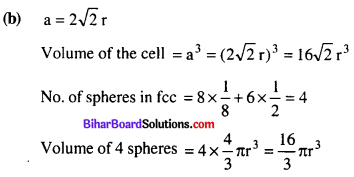Question 28.
Relationship between atomic radius and the edge length a of a body-centred cubic unit cell is ……..
(a) r = a/2
(b) $$r=\sqrt{a / 2}$$
(c) $$r=\frac{\sqrt{3}}{4} a$$
(d) $$r=\frac{3 a}{2}$$
(c) $$r=\frac{\sqrt{3}}{4} a$$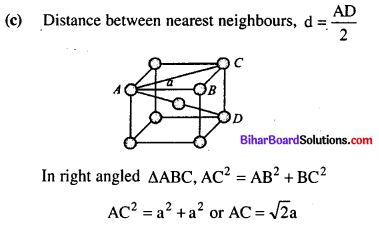Question 29.
Edge length of a unit cell of chromium metal is 287 pm with bcc arrangement. The atomic radius is of the order
(a) 124.27 pm
(b) 287 pm
(c) 574 pm
(d) 143.5 pm
(a) 124.27 pm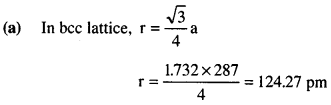Question 30.
The fraction of the total volume occupied by the atoms present in a simple cube is ………….
(a) $$\frac{\pi}{4}$$
(b) $$\frac{\pi}{6}$$
(c) $$\frac{\pi}{3 \sqrt{2}}$$
(d) $$\frac{\pi}{4 \sqrt{2}}$$
(b) $$\frac{\pi}{6}$$Question 31.
A unit cell of sodium chloride has four formula units. The edge length of the unit cell is 0.564 nm. What is the density of sodium chloride ?
(a) 3.89 g cm-3
(b) 2.16 gem-3
(c) 3 g cm-3
(d) 1.82 g cm-3
(b) 2.16 gem-3Question 32.
The distance between Na+ & Cl ions in NaCl with a density 3.165 g cm-3 is …………..
(a) 497 pm
(b) 248.5 pm
(c) 234 pm
(d) 538.5 pm
(b) 248.5 pm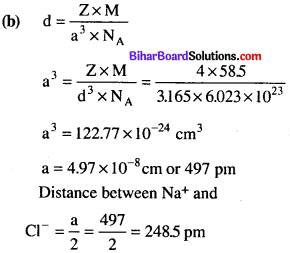Question 33.
How many lithium atoms are present in a unit cell with edge length 3.5A and density 0.53 g cm-3 ?
(a) 2
(b) 1
(c) 4
(d) 6
(a) 2Question 34.
Alkali halides do not show Frenkel defect because …………….
(a) cations and anions have almost equal size
(b) there is a large difference in size of cations and anions
(c) cations and anions have low coordination number
(d) anions cannot be accommodated in voids.
(a) cations and anions have almost equal size

Question 35.
Which of the following crystals does not exhibit Frenkel defect ?
(a) AgBr
(b) AgCl
(c) KBr
(d) ZnS
(c) KBr

Question 36.
What type of stoichiometric defect is shown by ZnS ?
(a) Schottky defect
(b) Frenkel defect
(c) Both Frenkel and Schottky defects
(d) Non-stoichiometric defect
(b) Frenkel defect

Question 37.
In a Schottky defect, …………..
(a) an ion moves to interstitial position between the lattice points
(b) electrons are trapped in a lattice site
(c) some lattice sites are vacant
(d) some extra cations are present in interstitial spaces.
(c) some lattice sites are vacantQuestion 38.
Silver halides generally show ………….
(a) Schottky defect
(b) Frenkel defect
(c) both Frenkel and Schottky defects
(d) cation excess defect.
(c) both Frenkel and Schottky defects

Question 39.
An electron trapped in an anion site in a crystal is called ………….
(a) F-centre
(b) Frenkel defect
(c) Schottky defect
(d) interstitial defect
(a) F-centre

Question 40.
Which of the following will have metal deficiency defect ?
(a) NaCl
(b) FeO
(c) KCl
(d) ZnO
(b) FeO

Question 41.
Pure silicon and germanium behave as …………
(a) conductors
(b) semiconductors
(c) insulators
(d) piezoelectric crystals
(c) insulators

Question 42.
To get n-type of semiconductor, germanium should be doped with ………….
(a) gallium
(b) arsenic
(c) aluminium
(d) boron
(b) arsenicQuestion 43.
p-type semiconductors are formed when Si or Ge are doped with …………
(a) group 14 elements
(b) group 15 elements
(c) group 13 elements
(d) group 18 elements.
(c) group 13 elements

Question 44.
Paramagnetic substances are magnetised in a magnetic field in the same direction. Paramagnetism is due to the presence of……………….
(a) one or more unpaired electrons
(b) all paired electrons
(c) permanent spin and orbital motion
(d) parallel and anti-parallel spins in equal numbers.
(a) one or more unpaired electrons

Question 45.
Fe3O4 is ferrimagnetic at room temperature but at 850 K, it becomes
(a) diamagnetic
(b) ferrimagnetic
(c) paramagnetic
(d) anti-ferromagnetic
(c) paramagnetic

Question 46.
Which of the following metal oxides is antiferromagnetic in nature ?
(a) MnO2
(b) TiO2
(c) NO2
(d) CrO2
(a) MnO2

Question 47.
Which of the following conditions favours the existance of a substance in the solid state ?
(a) High temperature
(b) Low temperature
(c) High thermal energy
(d) Weak cohesive forces
(b) Low temperatureQuestion 48.
Which of the following is an amorphous solid ?
(a) Graphite (c)
(b) Quartz glass (SiO2)
(c) Chrome alum
(d) Silicon carbide (SiC)
(b) Quartz glass (SiO2)

Question 49.
Iodine molecules are held in the crystals lattice by
(a) London forces
(b) dipole-dipole interactions
(c) covalent bonds
(d) coulombic forces
(a) London forces

Question 50.
Which of the following is a network solid ?
(a) SO2(Solid)
(b) I2
(c) Diamond
(d) H2O(Ice)
(c) Diamond

Question 51.
Graphite is a good conductor of electricity due to the presence of_______
(a) lone pair of electrons
(b) free valence electrons
(c) cations
(d) anions
(b) free valence electronsQuestion 52.
Which of the following oxides behaves as conductor or insulator depending upon temperature ?
(a) TiO
(b) SiO2
(c) TiO3
(d) MgO
(c) TiO3

Question 53.
Which of the following oxides shows electrical properties like metals ?
(a) SiO2
(b) MgO
(c) SO2(s)
(d) CrO2
(d) CrO2

Question 54.
The lattice site in a pure crystal cannot be occupied by
(a) molecule
(b) ion
(c) electron
(d) atom
(c) electron

Question 55.
Graphite cannot be classified as_____________ .
(a) conducting solid
(b) network solid
(c) covalent solid
(d) ionic solid
(d) ionic solid

Question 56.
Cations are present in the interstitial sites in___________
(a) Frenkel defect
(b) Schottky defect
(c) vacancy defect
(d) metal deficiency defect
(a) Frenkel defect

Question 57.
Which of the following is true about the charge acquired by p-type semiconductors ?
(a) positive
(b) neutral
(c) negative
(d) depends on concentration of p impurity
(b) neutralQuestion 58.
In which pair most efficient packing is present ?
(a) hep and bcc
(b) hep and ccp
(c) bcc and ccp
(d) bcc and simple cubic cell
(c) bcc and ccp

Question 59.
What is the coordination number in a square close packed structure in two dimensions ?
(a) 2
(b) 3
(c) 4
(d) 6
(c) 4

Question 60.
Which kind of defects are introduced by doping ?
(a) Dislocation defects
(b) Schottky defects
(c) Frenkel defects
(d) Electronic defects
(a) $$2 \sqrt{2} r, \frac{4 r}{\sqrt{3}}, 2 r$$
(b) $$\frac{4 r}{\sqrt{3}}, 2 \sqrt{2} r, 2 r$$
(c) $$2 \mathrm{r}, 2 \sqrt{2} \mathrm{r}, \frac{4 \mathrm{r}}{\sqrt{3}}$$
(d) $$2 \mathrm{r}, \frac{4 \mathrm{r}}{\sqrt{3}}, 2 \sqrt{2} \mathrm{r}$$
(a) $$2 \sqrt{2} r, \frac{4 r}{\sqrt{3}}, 2 r$$